Also called the'' Gaussian Quadrature or Legendre Quadrature. A Gaussian Quadrature over the interval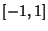with Weighting Function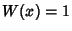. The Abscissas for quadrature orderare given by the roots of the Legendre Polynomials, which occur symmetrically about 0. The weights are(1)

whereis the Coefficient ofin. For Legendre Polynomials,(2)

so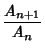(3)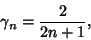(4)

so(5)

Using the Recurrence Relation(6)

gives(7)

The error term is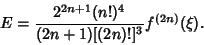(8)

Beyer (1987) gives a table of Abscissas and weights up to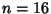, and Chandrasekhar (1960) up to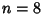forEven.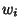2 ± 0.57735 1.000000 3 0 0.888889 ± 0.774597 0.555556 4 ± 0.339981 0.652145 ± 0.861136 0.347855 5 0 0.568889 ± 0.538469 0.478629 ± 0.90618 0.236927

The Abscissas and weights can be computed analytically for small.2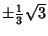1 3 0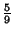4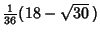References

Beyer, W. H. CRC Standard Mathematical Tables, 28th ed. Boca Raton, FL: CRC Press, pp. 462-463, 1987.

Chandrasekhar, S. Radiative Transfer. New York: Dover, pp. 56-62, 1960.

Hildebrand, F. B. Introduction to Numerical Analysis. New York: McGraw-Hill, pp. 323-325, 1956.